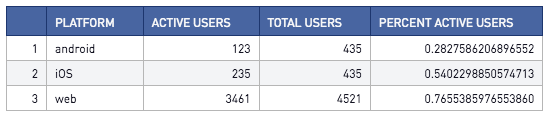# [Solution] SQL Division

Solution:

Multiply the numerator by 1.0

```select
platform
, active_users
, total_users
, active_users * 1.0 / total_users as percent_active_users
from
user_summary```Concepts Covered: Integer Division

Explanation:

In SQL, dividing 2 integers returns an integer. A quick way to resolve this is to multiply the numerator by 1.0. This divides a float by an integer, returning a float output.